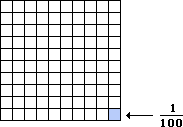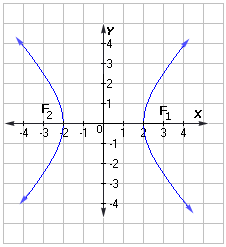Fraction

## Definition Of Hundredth

One out of one hundred equal parts is called a hundredth.The fractional form of one hundredth is 1/100 and the decimal equivalent is 0.01.
In a decimal, the second digit after the decimal point denotes hundredth. For example, there are seven hundredths in the number 2543.978.

### Solved Example on Hyperbola

#### Ques: Choose the standard form of the equation for the hyperbola shown.##### Choices:

A. 3
B. 4
C. 5
D. 6